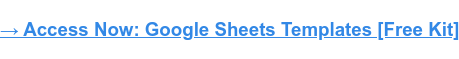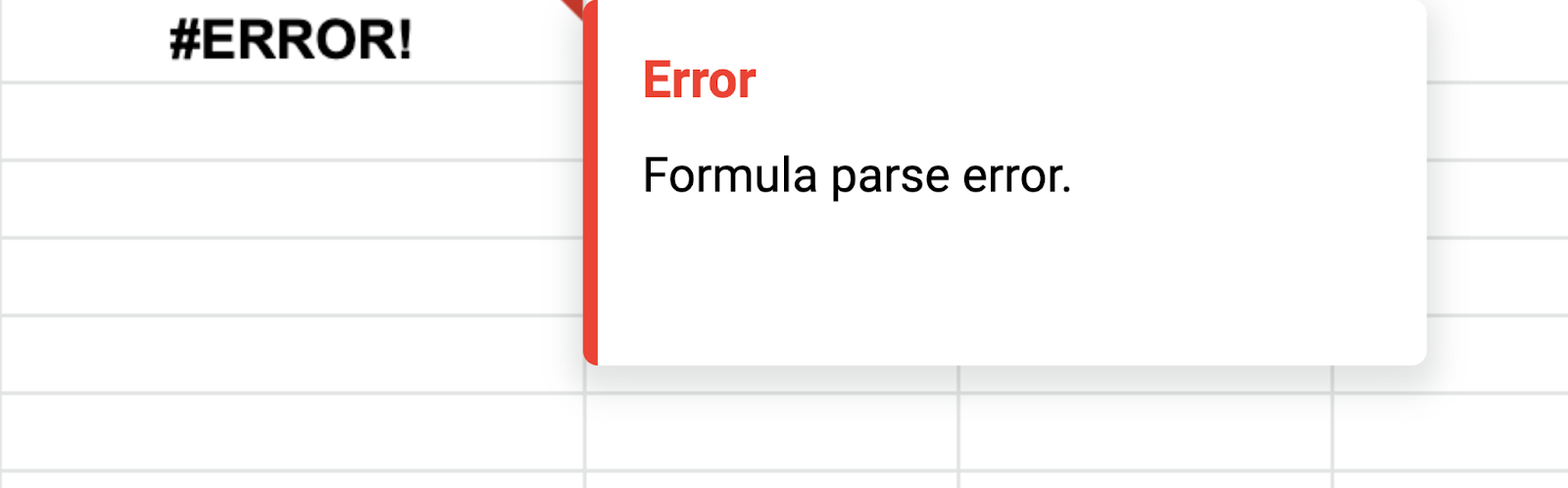# Formula Parse Errors: What They Are & How to Fix ThemPublished:

You're working in a spreadsheet and you want to use a function.You write the formula, excited to get the results, then you see "Formula parse error" leaving you feeling confused and a little defeated.

Let's cover what that actually means and what probably lead to that error message.

It's like trying to speak a different language without taking the time to learn it first.

The software can kind of make out what you're saying, but not well enough to give you an accurate result.There are two likely causes for this error: There's a typo in your formula, or the order of operations is unclear.

We'll go over some examples of each so that you can identify and fix them in your own formulas.

## Common Formula Parse Errors

Usually, a formula parse error happens because of:

Incorrect syntax – E.g.: Typing =+ instead of =, forgetting to put quotation marks around text values, putting two operators next to each other without anything in between them
Incomplete syntax – E.g. Leaving out a parentheses.

Another reason why you may be getting these errors is that you’re trying to use text values where numbers are expected.

Let’s dive into the specific types of errors you may encounter:

### #N/A Error

One of the most common errors is the #N/A error. It occurs when a formula can't find what it's looking for.

For example, if you're using the VLOOKUP function to find a value in a table, and the value you're looking for isn't in the table, you'll get the #N/A error.

### #DIV/0 Error

This happens when you try to divide a number by zero.

For example, if you have a formula =A17/B17 and the value in B17 is 0, you'll get the #DIV/0! error.

### #REF! Error

When a formula contains an invalid cell reference, you will get this error message.

For example, if you have a formula that references cells A17:A22 and you delete row 21, the formula will return the #REF! error because it no longer has a valid reference.

### #VALUE Error

The #VALUE! error occurs when a formula contains an invalid value.

For example, if you have a formula that multiplies two cells and one of the cells contains text instead of a number, you'll get this error.

### #NAME Error

This error occurs when a formula contains an invalid name.

For example, if you have a named range called " Prices" and you accidentally type "price" in your formula, you'll get the #NAME? error.

### #NUM Error

The #NUM! error occurs when a formula contains an invalid number.

Say you have a formula that divides two cells and the result is too large to be displayed, you'll get this error.

Now that we know what can cause a formula parse error, let's look at how we can fix them.

## How to Fix Formula Parse Errors

The best way to avoid getting formula parse errors is to carefully check your syntax as you type it out. If you're not sure what order the operations should go in, refer back to the order of operations suggested by the software you’re using.

If you're getting formula parse errors, here are some steps you can take to fix them:

• Check your formula inputs and make sure they're correct.
• Use the IFERROR function and display a different result if an error occurs. E.g. "Not found."
• Check your spelling and make sure all the parentheses are in the right places.
• Make sure you're using the correct operators.
• Use cell references instead of hard coding values into your formulas.
• If you're using text values, make sure they're enclosed in quotation marks.

By following these steps, you can avoid formula parse errors and get accurate results from your formulas.